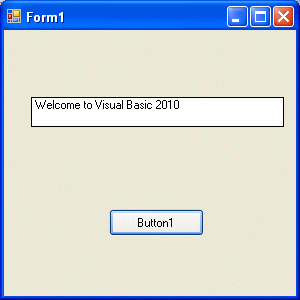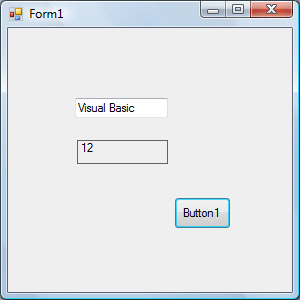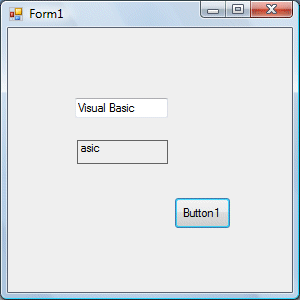># Lesson 8 : String Manipulation

String manipulation is an important part of programming because it help to process data that come in the form of non-numeric types such as name, address, city, book title and more.

### 8.1 String Manipulation Using + and & signs.

Strings can be manipulated using the & sign and the + sign, both perform the string concatenation which means combining two or more smaller strings into larger strings. For example, we can join "Visual" and "Basic" into "Visual Basic" using "Visual"&"Basic" or "Visual "+"Basic", as shown in the example below

#### Example 8.1(a)

```Private Sub Button1_Click(ByVal sender As System.Object, ByVal e As System.EventArgs) Handles Button1.Click
Dim text1, text2, text3 As String
text1 = "Visual"
text2 = "Basic"
text3 = text1 + text2
Label1.Text = text3
End Sub
```

The line text3=text1+ text2 can be replaced by text3=text1 & text2  and produced the same output. However, if one of the variables is declared as numeric data type, you cannot use the + sign, you can only use the & sign.

#### Example 8.2

```Dim text1, text3 as string
Dim Text2 As Integertext1 = "Visual"
text2=22
text3=text1+text2
Label1.Text = text3
```

This code will produce an error because of data mismatch.However, using & instead of + will be all right.

```Dim text1, text3 as string
Dim Text2 As Integer
text1 = "Visual"
text2=22
text3=text1 & text2
Label1.Text = text3
```

You can combine more than two strings to form a larger strings, like the following example:

```Public Class Form1
Private Sub Button1_Click(ByVal sender As System.Object, ByVal e As System.EventArgs) Handles Button1.Click
Dim text1, text2, text3, text4, text5, text6 As String
text1 = "Welcome"
text2 = " to"
text3 = " Visual"
text4 = " Basic"
text5 = " 2010"
text6 = text1 + text2 + text3+text4+text5
Label1.Text = text6
End Sub
End Class
```

Running the above program will produce the following screen shot, as shown in Figure 8.1### 8.2 String Manipulation Using VB2010 Built-in Functions

A function is similar to a normal procedure but the main purpose of the function is to accept a  certain input and return a value which is passed on to the main program to finish the execution.There are numerous string manipulation functions built into VB2010 but I will only discuss a few here and will explain the rest of them in later Lessons.

#### 8.2 (a) The Len Function

The length function returns an integer value which is the length of a phrase or a sentence, including the empty spaces. The format is

`Len ("Phrase")`

For example,

#### Example 8.3

```Public Class Form1
Private Sub Button1_Click(ByVal sender As System.Object, ByVal e As System.EventArgs) Handles Button1.Click
Label1.Text = Len(TextBox1.Text)
End Sub
End Class
```

The output is shown in Figure 8.2#### 8.2(b) The Right Function

The Right function extracts the right portion of a phrase. The syntax is

` Microsoft.VisualBasic.Right("Phrase",n)`

#### Example 8.3

```Private Sub Button1_Click(ByVal sender As System.Object, ByVal e As System.EventArgs) Handles Button1.Click
Dim text1 As String
text1 = TextBox1.Text
Label1.Text = Microsoft.VisualBasic.Right(text1, 4)
End Sub
```

The above program will return four right most characters of the phrase entered into the textbox.

The Outputis shown in Figure 8.3#### Figure 8.3

*The reason of using the full reference is because many objects have the Right properties so using Right on its own will make it ambiguous to VB2010.

#### 8.2(c)The Left Function

The Left function extract the left portion of a phrase. The format is

```
Microsoft.VisualBasic.Left("Phrase",n)```

Where n is the starting position from the left of the phase where the portion of the phrase is going to be extracted.  For example,

`Microsoft.VisualBasic.Left ("Visual Basic", 4) = Visu`